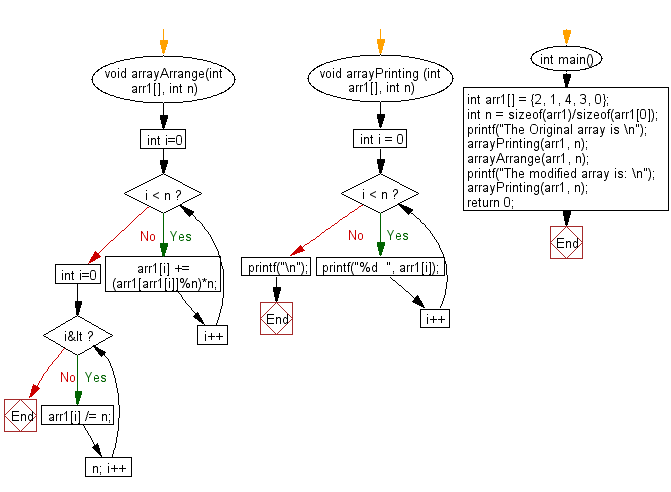﻿ C exercises: Rearrange an array so that arr[i] becomes arr[arr[i]] from an array of size n and elements are in the range 0 to n-1 - w3resource# C Exercises: Rearrange an array so that arr[i] becomes arr[arr[i]] from an array of size n and elements are in the range 0 to n-1

## C Array: Exercise-90 with Solution

Given an array of size n such that every element is in the range from 0 to n-1. Write a program in C to rearrange the given array so that arr[i] becomes arr[arr[i]].

Sample Solution:

C Code:

``````#include<stdio.h>

void arrayArrange(int arr1[], int n)
{
for (int i=0; i < n; i++)
arr1[i] += (arr1[arr1[i]]%n)*n;

for (int i=0; i<n; i++)
arr1[i] /= n;
}

void arrayPrinting (int arr1[], int n)
{
for (int i = 0; i < n; i++)
printf("%d  ", arr1[i]);
printf("\n");
}

int main()
{
int arr1[] = {2, 1, 4, 3, 0};
int n = sizeof(arr1)/sizeof(arr1);

printf("The Original array is \n");
arrayPrinting(arr1, n);

arrayArrange(arr1, n);

printf("The modified array is: \n");
arrayPrinting(arr1, n);
return 0;
}
```
```

Sample Output:

```The Original array is
2  1  4  3  0
The modified array is:
4  1  0  3  2
```

Flowchart:C Programming Code Editor:

Improve this sample solution and post your code through Disqus.

﻿